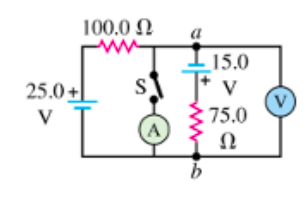# Problem: In the circuit shown in the figure (Figure 1) all meters are idealized and the batteries have no appreciate interal resistance.Part A. With the switch S open which point is at a higher potential: a or b?a. point ab. point bPart B. Find the reading of the voltmeter with the switch S open.Va – Vb =Part C. With the switch closed, find the reading of the voltmeter.Part D. With the switch closed, find the reading of the ammeter.

###### FREE Expert Solution

Kirchhoff's voltage law:

$\overline{){\mathbf{\Sigma }}{\mathbf{V}}{\mathbf{=}}{\mathbf{0}}}$

From Ohm's law:

$\overline{){\mathbf{V}}{\mathbf{=}}{\mathbf{i}}{\mathbf{R}}}$, where V is voltage, i is current, and R is resistance.

Part A

Using Kirchhoff's voltage law on the left loop in a clockwise direction:

$\begin{array}{rcl}\mathbf{25}\mathbf{-}\mathbf{100}\mathbf{i}\mathbf{+}\mathbf{15}\mathbf{-}\mathbf{i}\mathbf{75}& \mathbf{=}& \mathbf{0}\\ \mathbf{40}\mathbf{-}\mathbf{i}\mathbf{175}& \mathbf{=}& \mathbf{0}\\ \mathbf{i}\mathbf{175}& \mathbf{=}& \mathbf{40}\\ \mathbf{i}& \mathbf{=}& \frac{\mathbf{40}}{\mathbf{175}}\end{array}$

85% (183 ratings)###### Problem Details

In the circuit shown in the figure (Figure 1) all meters are idealized and the batteries have no appreciate interal resistance.Part A. With the switch S open which point is at a higher potential: a or b?
a. point a
b. point b

Part B. Find the reading of the voltmeter with the switch S open.
Va – Vb =

Part C. With the switch closed, find the reading of the voltmeter.

Part D. With the switch closed, find the reading of the ammeter.